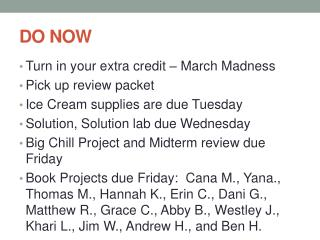# DO NOW - PowerPoint PPT PresentationDownload PresentationDO NOW

DO NOWDownload Presentation## DO NOW

- - - - - - - - - - - - - - - - - - - - - - - - - - - E N D - - - - - - - - - - - - - - - - - - - - - - - - - - -
##### Presentation Transcript

1. DO NOW • Turn in your extra credit – March Madness • Pick up review packet • Ice Cream supplies are due Tuesday • Solution, Solution lab due Wednesday • Big Chill Project and Midterm review due Friday • Book Projects due Friday: Cana M., Yana., Thomas M., Hannah K., Erin C., Dani G., Matthew R., Grace C., Abby B., Westley J., Khari L., Jim W., Andrew H., and Ben H.

2. SOLUTIONS A Review

3. HOMEWORK CHECK – ver. 1 1. Calculate the molarity of the following solutions: a. 800.0 mL which contains 30.0 g of acetic acid (CH3COOH) 4. Calculate the molality of the following: b. 3.00 mol H2SO4 dissolved in 1250.0g water 6a. What is the mass percent of the solute and solvent if there is 7.590g glucose (C6H12O6) dissolved in 125g water? 8a. What is the freezing point of a solution that contains 10.0 g naphthalene, C10H8, dissolved in 50.0 g benzene, C6H6? (The Kfp of benzene is 5.12C/m and the freezing point is 5.533C.)

4. HOMEWORK CHECK – ver. 2 1. Calculate the molarity of the following solutions: b. 2000.0 mL which contains 49.0 g of phosphoric acid, H3PO4. 3c. How many liters of solution can be made from 1.00M solution using 50.0g copper (II) sulfate pentahydrate? 7a. What is the volume percent for the solute and solvent if there is 35 mL ethanol dissolved in 115mL water? 8a. What is the boiling point of a solution that contains 10.0 g naphthalene, C10H8, dissolved in 50.0 g benzene, C6H6? (The Kbp of benzene is 2.53C/m and the boiling point is 80.100C.)

5. HOMEWORK CHECK – ver. 3 1. Calculate the molarity of the following solutions: c. 250.0 mL which contains 50.0 g of copper (II) sulfate pentahydrate, CuSO4• 5H2O. 4a. Calculate the molality if there is 1.50 mol NaCH3COO dissolved in 750.0g water. 5. What are the mole fractions for solute and solvent if there are 7.590g glucose, C6H12O6 dissolved in 125g water? 8b. What is the freezing point of a solution that contains 23.0 g MgSO4 dissolved in 250.0 g water? (The Kfp of water is 1.853C/m .)

6. SOLUTIONS PROBLEM SET • Get out homework to go over.

7. REVIEW • Differentiate between colloids, solutions, and suspensions • Define solvent and solute and be able to identify them in a solution. • Describe the process of solvation, dissociation, and dissolving. • Be sure to study polarity of compounds to be able to predict solubility. • Differentiate between saturated, unsaturated, and supersaturated.

8. REVIEW • State and discuss the factors affecting the rate of solubility. • State and discuss the factors that affect solubility. • Be able to read a solubility curve graph. • Relate the enthalpy of solution to endothermic and exothermic dissolving processes. • Differentiate between molarity and molality. • Solve problems involving molarity, molality, mole fraction, mass percent, volume percent, and making solutions.

9. Solubility Go back and look over handout and make sure you can answers questions like to ones on the handout.

10. REVIEW • Describe the three colligative properties of solutions (vapor pressure reduction, freezing point depression, and boiling point elevation). • Relate the colligative properties to everyday situations. • Calculate the freezing point, boiling point and molar mass using changes in temperature and molality.

11. THE TEST • 12 multiple choice • 6 problems • 2 short answer • Solubility graph with four questions

12. THE TEST Potential Problems: • Molarity (M, grams, liters) • Molality (m, grams, kilograms) • Mole Fraction, Mass Percent, volume percent • Making a solution (acid and solids) • Boiling pt elevation or freezing pt depression • Molar Mass calculation from ΔT

13. GIVEN • I will give you all constants, boiling points and freezing points (except water’s boiling and freezing points). • I will NOT give you any formulas, • You will have a periodic table.